Courses

# Test: Dimensions of Unit Cells

## 10 Questions MCQ Test Chemistry for JEE | Test: Dimensions of Unit Cells

Description
This mock test of Test: Dimensions of Unit Cells for JEE helps you for every JEE entrance exam. This contains 10 Multiple Choice Questions for JEE Test: Dimensions of Unit Cells (mcq) to study with solutions a complete question bank. The solved questions answers in this Test: Dimensions of Unit Cells quiz give you a good mix of easy questions and tough questions. JEE students definitely take this Test: Dimensions of Unit Cells exercise for a better result in the exam. You can find other Test: Dimensions of Unit Cells extra questions, long questions & short questions for JEE on EduRev as well by searching above.
QUESTION: 1

### If an element crystallizes as a simple cube, what is the volume of an element provided its density is 1.5 g/cm3 and atomic mass of the element is 63?

Solution:

Simple cube has 1 atom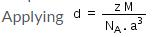Therefore volume of cube i.e. a3 = 6.97 x 10-23 cm3

QUESTION: 2

### Mass of an atom in an unit cell is calculated by which of the following formula if M is the molar mass:

Solution:

(Molar mass = M) (1 molar of atom = M 6.023×1023)( atom = M/Na)

QUESTION: 3

### In the formula to calculate the density of a unit cell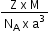what is z ?

Solution:

The correct answer is Option B.
Mass of unit cell = number of atoms in unit cell × mass of each atom = z × m
Where, z = number of atoms in unit cell,
m = Mass of each atom
Mass of an atom can be given with the help of Avogadro number and molar mass as: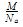Where, M = molar mass
NA = Avogadro’s number
Volume of unit cell, V = a3
⇒ Density of unit cell =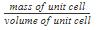⇒ Density of unit cell =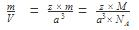QUESTION: 4

Volume of a body centred cubic unit cell is

Solution:

In a body centered cubic unit cell ,√3a = 4r a= 4r/√3 where a = side of the cube volume = a3 = (4r/√3)3.

QUESTION: 5

Density of a unit cell is equal to mass of unit cell divided by

Solution:

Volume of unit cell, V = a3

=> Density of unit cell = mass of unit cell /volume of unit cell

QUESTION: 6

Length a in unit cell is along

Solution:

The correct answer is option A
Unit cell is essentially considered a cube, so a cube has 3 axes. X,Y,Z so X represents length , Y represents breadth, Z represents height.

QUESTION: 7

The number of atoms (z) in simple cubic unit cell is

Solution:

The face-centered cubic (fcc) has a coordination number of 12 and contains 4 atoms per unit cell. The body-centered cubic (bcc) has a coordination number of 8 and contains 2 atoms per unit cell. The simple cubic has a coordination number of 6 and contains 1 atom per unit cell.

QUESTION: 8

X-ray diffraction studies show that copper crystallizes in an fcc unit cell with cell edge of 3.608 x 10-8 cm. In a separate experiment, copper is determined to have a density of 8.92 g/cm3, calculate the atomic mass of copper.

Solution:

In case of fcc lattice,number of atoms per unit cell,x=4 atoms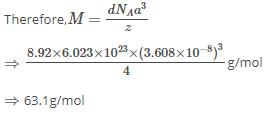∴  Atomic mass of copper =63.1u

QUESTION: 9

An element with bcc geometry has atomic mass 50 and edge length 290 pm. The density of unit cell will be

Solution:

Length of the edge , a = 290 pm =290 x 10-10 cm
Volume of unit cell = ( 290 x 10-10 cm )3 = 24.39 x 10-24 cm3

Since it is bcc arrangement,
Number of atoms in the unit cell, Z = 2
Atomic mass of the element = 50

Mass of the atom = atomic mass/ Avogadro number = M/No = 50/6.02 x 1023

Mass of the unit cell = Z x M/No = 2 x 50/6.02 x 1023 = 100/6.23 x 1023
Therefore , density = mass of unit cell / volume of unit cell
= 100/6.023 x 1023 x 24.39 x 10-24 = 6.81 g cm-3

QUESTION: 10

The number of octahedral void(s) per atom present in a cubic close-packed structure is

Solution:

The total number of octahedral void(s) per atom present in a cubic close packed structure is 4. Besides the body centre, there is one octahedral void at the centre of each of the 12 edges. It is surrounded by six atoms, four belonging to the same unit cell (2 on the corners and 2 on face centres) and two belonging to two adjacent unit cells. Since each edge of the cube is shared between four adjacent unit cells, so is the octahedral void located on it. Only 1/4 ​th of each void belongs to a particular unit cell. Thus, in cubic close packed structure, octahedral void at the body-centre of the cube is 1.
12 octahedral voids located at each edge and shared between four unit cells=12× 1/4​=3
Total number of octahedral voids =4
We know that in ccp structure, each unit cell has 4 atoms. Thus, the number of octahedral voids is equal to 4/4=1.